V1

2022/09/22阅读：37主题：蔷薇紫

# JS算法之二叉树、二叉搜索树

### 你能所学到的知识点

1. 知识点简讲
• 树在前端开发中的应用场景
• 二叉树深度优先遍历 递归和迭代的JS版本
2. 二叉树相关算法
3. 二叉搜索树（`BST`）相关算法

# 知识点简讲

## 树的简介

• 在编译程序中，用树来表示源程序的语法结构

• `babel`进行代码编译的时候，在中间过程(`Trasnfrom`)中就会生成代表源码代码的`AST`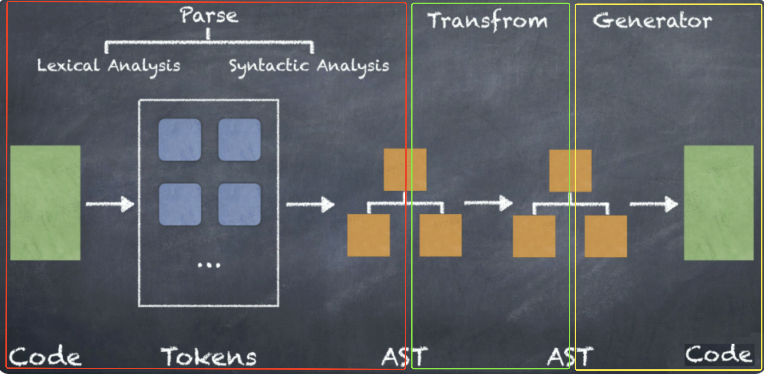• 在前端框架(`Vue/React`)中，用`element树`来表示即将被渲染到页面的数据信息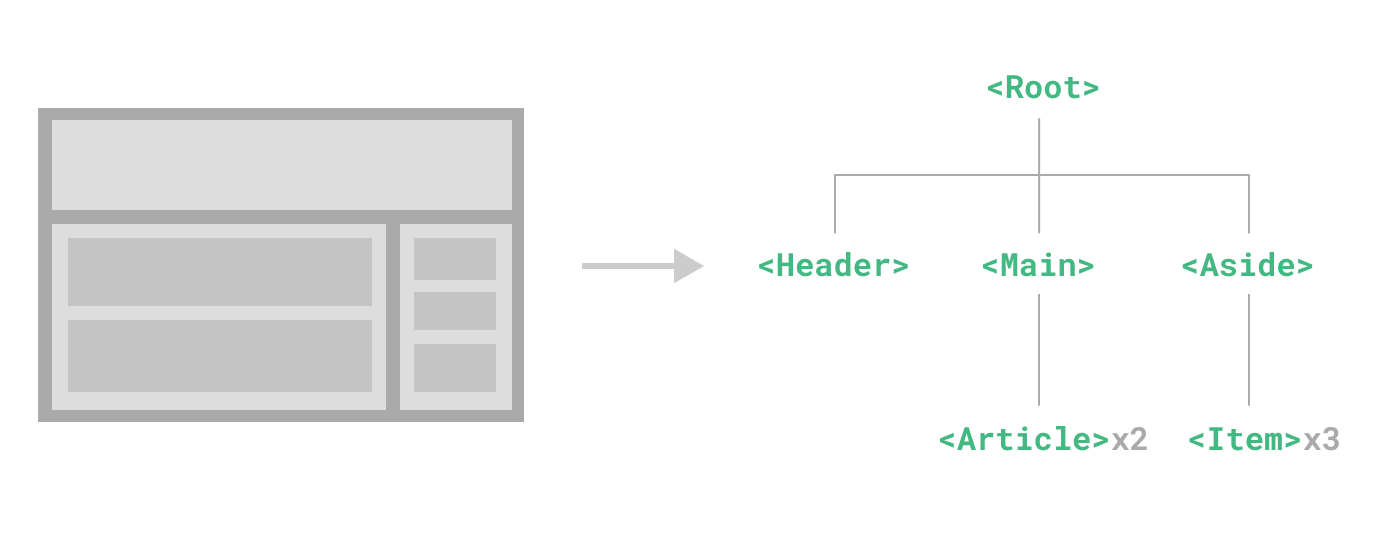• `React`最新的`Fiber`框架中，还拥有一棵`Fiber`树，用于保存针对页面的副作用。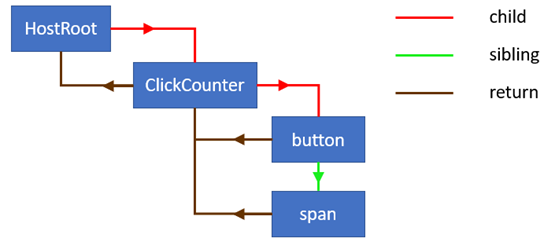• 在数据库系统中，可用树来组织信息；

• 在分析算法的行为时，可用树来描述其执行过程等等。

• 有且仅有一个特定的称为根（`Root`）的结点；
• `n>1`时，其余结点可分为`m(m>0)`互不相交的有限集`T1,T2,T3,...Tm`，其中每一个集合本身又是一棵树，并且称为根的子树（`Subtree`）。

## 二叉树和二叉搜索树

• 一个是左侧子节点，
• 另一个是右侧子节点

• 只允许你在左侧节点存储（比父节点）小的值
• 在右侧节点存储（比父节点）大的值

### 二叉树的数据结构

`Node` 类来表示二叉树中的每个节点，代码如下。

``class Node { constructor(key) {   this.key = key; // {1} 节点值   this.left = null; // 左侧子节点引用   this.right = null; // 右侧子节点引用 }} ``

## 二叉树的遍历

1. 广度优先遍历Breath-First-Search - `BFS`
• 我们在队列中有过相关介绍，这里就不在赘述了。
2. 深度优先遍历Depth-First-Search - `DFS`
`DFS`又根据遍历根节点的先后顺序，分为
1. 中序遍历Inorder Traversal
• 遍历左子树–>访问根–>遍历右子树;
1. 前序遍历Preorder Traversal
• 访问根–>遍历左子树–>遍历右子树;
1. 后序遍历Postorder Traversal
• 遍历左子树–>遍历右子树–>访问根;

### 中序遍历Inorder Traversal

#### 递归版本

``function inOrderTraverse(root) {    if (root == null) return;     // 基线条件    inOrderTraverse(root.left);    console.log(root.data + " ");    inOrderTraverse(root.right);}``

#### 迭代版本

• 二叉树的遍历总是从根节点开始的，但当第一次到达根节点时，并不是马上遍历根节点，而是顺着指向左子节点的指针向下直到叶子节点，也就是找到第一个真正被遍历的节点
• 为了在一个节点被遍历之后能够接着回去遍历它的父节点
• 可以在顺着指向左子节点的指针遍历二叉树时，把遇到的每个节点都添加到一个栈中
• 当一个节点被遍历之后，就可以从栈中得到它的父节点
``function inOrderTraverse(root) {    let result = [];    let stack = new Stack();  // 这里的Stack()在前面的文章中有介绍    let cur = root;    while(cur || !stack.isEmpty()) {         while(cur){          stack.push(cur);          cur = cur.left;        }        cur = stack.pop();        result.push(cur.val); // 操作当前节点        cur = cur.right;     }    return result;}``

• `cur`表示当前遍历的节点。
• 如果该节点有左子节点，按照中序的遍历顺序，应该先遍历它的左子树。
• 指向左子节点的指针一直向下移动，并将沿途遇到的每个节点都添加到栈中
• `while(cur){ stack.push(cur); cur = cur.left; }`
• 第二个`while`结束之后，最左子节点位于栈顶

### 前序遍历Preorder Traversal

#### 递归版本

``// 先序遍历function preOrderTraverse(root) {    if (root == null)  return;    // 基线条件    console.log(root.data + " ");    preOrderTraverse(root.left);    preOrderTraverse(root.right);}``

#### 迭代版本

``function preOrderTraverse(root) {    let result = [];    let stack = new Stack();  // 这里的Stack()在前面的文章中有介绍    let cur = root    while(cur || !stack.isEmpty()) {         while(cur){          result.push(cur.val); // 操作当前节点          stack.push(cur);          cur = cur.left;        }        cur = stack.pop();        cur = cur.right;    }    return result;}``

``function xxOrderTraverse(root) {    let result = [];    let stack=new Stack();      let cur = root    while(cur || !stack.isEmpty() ) {         while(cur){+         result.push(cur.val); // 中序遍历          stack.push(cur);          cur = cur.left;        }        cur = stack.pop();+       result.push(cur.val); // 前序遍历        cur = cur.right;    }    return result;}``

### 后序遍历Postorder Traversal

#### 递归版本

`` function postOrderTraverse(root) {    if (root == null) return;      // 基线条件    postOrderTraverse(root.left);    postOrderTraverse(root.right);    console.log(root.data + " ");}``

#### 迭代版本

• 如果之前还没有遍历过它的右子树就的前往它的右子节点
• 如果之前已经遍历过它的右子树那么就可以遍历当前节点

``function postOrderTraverse(root) {    let result = [];    let stack = new Stack();    let cur = root;        let prev = null;// 记录前一次被访问的节点信息    while(cur || !stack.isEmpty()) {       while(cur){         stack.push(cur);         cur = cur.left;       }              cur = stack.peek();       if(cur.right && cur.right != prev){         // 一个节点存在右子树且未被遍历         cur = cur.right;       } else {         stack.pop();         result.push(cur.val);         prev = cur;         cur = null;       }     }    return result; }``

• `prev`就是遍历过的前一个节点，它的初始值为`null`
• 在准备遍历下一个节点之前，就把它指向当前遍历的节点
• `prev = cur; cur = null;`
• `cur`表示当前到达的节点。
• 如果该节点有右子树并且右子节点不是前一个遍历的节点，则表示它有右子树并且右子树还没有遍历过
• `cur.right && cur.right != prev`

### 小结

• 它们都需要一个栈
• `stack = new Stack()`
• 基本结构也很相像
• 都有两个`while`循环并且它们的条件都一样
• 第一个`while`
• `while(cur || !stack.isEmpty())`
• 当前元素非空 或者 栈非空
• 第二个`while`
• `while(cur)`
• 当前元素非空
• 遍历当前节点的时机
• 前序遍历：
• 一边顺着指向左子节点的指针移动一边遍历当前的节点
•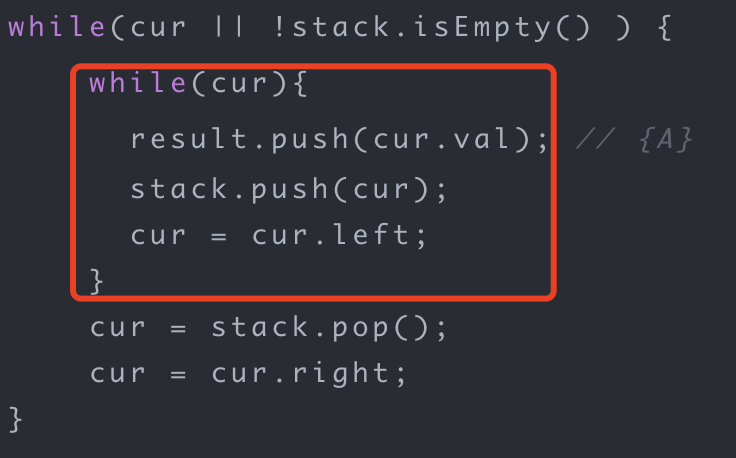• 中序遍历和后序遍历：
• 顺着指向左子节点的指针移动时，只将节点放入栈中，并不遍历遇到的节点
• 只有当到达最左子节点之后再从栈中取出节点遍历
•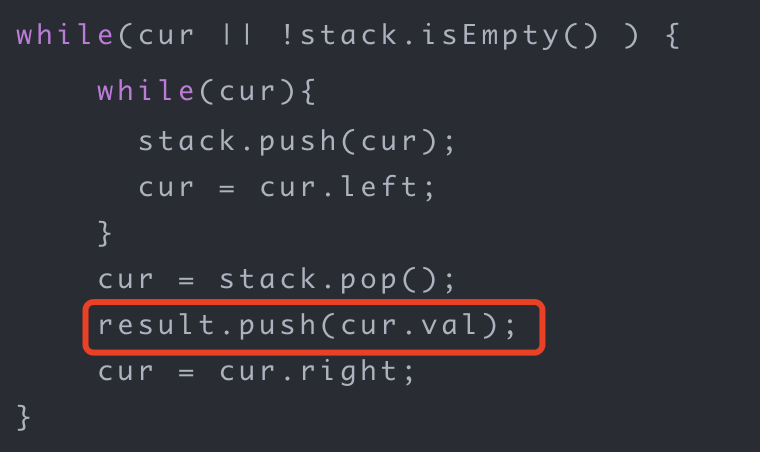• 后序还需要保存前一个遍历的节点，并根据前一个遍历的节点是否为当前节点的右子节点来决定此时是否可以遍历当前节点
•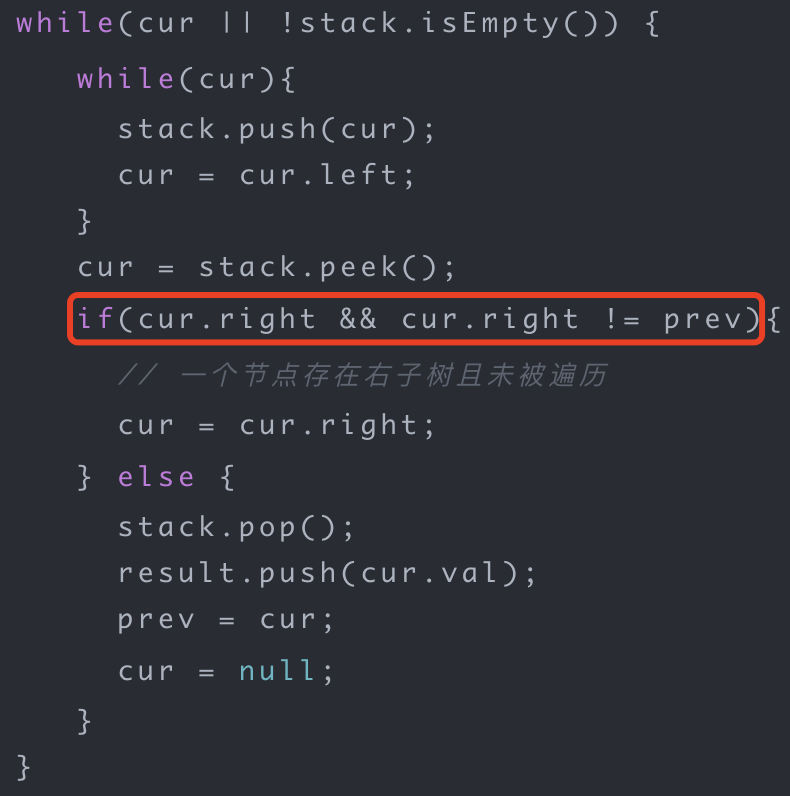# 二叉树相关算法

## 二叉树剪枝### 分析

1. 什么样的节点可以被删除
• 首先，这个节点的值为`0`
• 其次，如果它有子树，那么它的子树的所有节点都可以被删除
2. 后序遍历适合处理这个问题
• 用后序遍历的顺序遍历到某个节点，那么它的左右子树的节点一定已经遍历过了

### 代码实现

``function pruneTree(root){  if(root == null) return root; // 基线条件    root.left = pruneTree(root.left);  root.right = pruneTree(root.right);  if(root.left == null     && root.right == null     && root.val == 0){      return null    }  return root;}``

• 每当遍历到一个节点，就要确定它是否有左右子树，
• 如果左右子树都是空，并且节点的值是0
• 那么就可以删除这个节点
• 所谓删除一个节点，就是返回`null`给它的父节点
• `return null`

## 从根节点到叶节点的路径数字之和

• 从根到叶子节点路径 4->9->5 代表数字 495
• 从根到叶子节点路径 4->9->1 代表数字 491
• 从根到叶子节点路径 4->0 代表数字 40
因此，数字总和 = 495 + 491 + 40 = 1026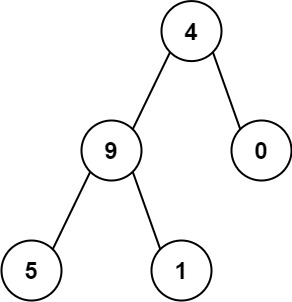### 分析

1. 顺着指向子节点的指针路径向下遍历二叉树，每到达一个节点，相当于在路径表示的数字末尾添加一位数字
• 最开始到达根节点`4`，然后到达节点`9`,此时路径表示的数字`49 = 4x10 + 9`
• 然后向下到达节点`5`,此时路径表示的数字`495` (`49 x10 + 5`)
2. 每当遍历到一个节点时都计算从根节点到当前节点的路径表示的数字。
• 如果这个节点还有子节点，就把值传下去继续遍历子节点
• 先计算当前节点，再计算子节点 --> 前序遍历

### 代码实现

``function sumNumbers(root){  return (function dfs(root,path){    if(root == null) return 0;  // 基线条件    path = path * 10 + root.val;    // 如果是叶子节点，返回对应的值    if(root.left ==null && root.right == null){      return path;    }    // 有子节点，把值path向下传递    return dfs(root.left,path) + dfs(root.right,path)  })(root,0)}``

• 路径定义是从根节点开始到叶节点结束，因此只有遇到叶节点才返回路径表示的数字
• `if(root.left) ==null && root.right ==null) return path`
• 在遇到叶节点之前就结束的路径，应该返回0
• 如果在某个非叶子节点，不存在左子树，那当遍历左子树时，此时值为`null`,如果从中获取节点的值`xx.val`就会报错。所以针对这种情况需要做一个容错处理
• `if(root == null) return 0`;

## 向下的路径节点值之和

### 分析

1. 路径的起止节点的不确定性给计算路径经过的节点值之和带来了很大的难度
• 虽然路径不一定从根节点开始，但仍然可以求得从根节点开始到达当前遍历节点的路径所经过的节点值之和
2. 在路径上移动时把所有累加的节点值之和都保存下来，就容易知道是否存在从任意节点出发的值为给定sum的路径
3. 当遍历到一个节点时，先累加从根节点开始的路径上的节点值之和，再计算到它的左右子节点的路径的节点值之和。
• 采用前序遍历

### 代码实现

``function pathSum(root,sum){  let sumToCount = new Map();  sumToCount.set(0,1);  return (function dfs(root,sum,sumToCount,path){    if(root ==null) return 0;        path+=root.val;    let count = sumToCount.get(path -sum) || 0;    sumToCount.set(path,(sumToCount.get(path)||0)+1);        count += dfs(root.left,sum,sumToCount,path);    count += dfs(root.right,sum,sumToCount,path);        sumToCount.set(path,sumToCount.get(path) -1);        return count;  })(root,sum,sumToCount,0)}``

• `path`表示从根节点开始的路径已经累加的节点值之和，并保存到哈希表`sumToCount`
• 初始值为`sumToCount.set(0,1)` ==> `sum``0`的个数为`1`
• `sumToCount`
• 是累加的节点值之和
• 是每个节点值之和出现的次数
• 当遍历到一个节点时，就把当前的节点值累加到`path`
• `path += root.val`
• 在已保存的路径前缀和中查找是否存在前缀和刚好等于当前节点到根节点的前缀和 `path` 减去 `sum`
• 如果这个和之前出现过(`sumToCount.get(path -sum)`存在)，则将出现的次数+1
• 如果不存在，`count = 0`
• 更新哈希表`sumToCount.set(xx,xx)`
• 累加节点值之和`path`
• path出现的次数
• 当递归函数`dfs`结束时，程序将回到节点的父节点，也就是说，在函数结束之前需要将当前节点从路径中删除，从根节点到当前节点累加的节点值之和也要从哈希表`sumToCount`中删除
• `sumToCount.set(path,sumToCount.get(path) -1);`

# 二叉搜索树（BST）

``function searchBST(root,val){  let cur = root;  while(cur){    if(cur.val == val){      break;    }        if(cur.val < val){      cur = cur.right;    }else{      cur = cur.left;    }  }  return cur;}``

## 展开二叉搜索树

### 分析

1. 需要按照节点的值递增的顺序遍历二叉搜索树中的每个节点，并将节点用指向右子节点的指针连接起来。
2. 采用中序遍历，只是在每遍历到一个节点要把前一个节点的指向右子节点的指针指向当前节点

### 代码实现

``function increasingBST(root){  let stack = new Stack();  let cur = root;  let prev = null;  let first = null;  while(cur || !stack.isEmpty()){    while(cur){      stack.push(cur);      cur = cur.left;    }        cur = stack.pop();    if(prev){      prev.right = cur;    }else {      first = cur;    }        prev = cur;    cur.left = null;    cur = cur.right;  }  return first;}``

• 变量`prev`表示前一个遍历到的节点
• 当遍历到当前节点`cur`时，
• 把变量`prev`右子节点的指针指向`cur`
• `prev.right = cur`
• 并将`cur`指向左子节点的指针设为`null`
• `cur.left = null`
• 展平之后的二叉搜索树的根节点是值最小的节点，也是中序遍历第一个被遍历到的节点。我们用`first`来保存这个信息
• `prev``null``first = cur`

## 二叉搜索树的下一个节点

### 时间复杂度O(n)的解法

#### 分析

1. 采用二叉树的中序遍历
2. 用一个布尔值`found`来记录已经遍历到的节点p
• 初始化为`false`
• 遍历到节点`p`就将它设为`true`
• 在这个变量变成`true`之后遍历到的第一个节点就是要找的节点
``function inorderSuccessor(root,p){  let stack = new Stack();  let cur = root;  let found = false;  while(cur || !stack.isEmpty()){    while(cur){      stack.push(cur);      cur = cur.left;    }        cur = stack.pop();        if(found){      break;    }else if(cur == p){      found = true;    }    cur = cur.right;  }  return cur;}``

### 时间复杂度O(h)的解法

1. 在中序遍历的情况下，下一个节点的值一定不小于节点`p`的值，而且还是大于或等于节点`p`的值的所有节点中最小的一个
2. 从根节点开始，每到达一个节点就比较子树根节点的值和节点`p`的值。
• 如果当前节点的值小于或者等于`p`的值，那么节点`p`的下一个节点应该在它的右子树
• 如果当前节点的值大于节点`p`的值，那么当前节点有可能是它的下一个节点。
• 此时当前节点的值比节点`p`的值大，但节点`p`下一个节点是所有比它大的节点中值最小的一个
• 接下来前往当前节点的左子树，确定是否能找到值更小但仍然大于节点`p`的值的节点
• 重复这样的比较，直到找到最后一个大于节点`p`的值的节点，就是节点`p`的下一个节点
``function inorderSuccessor(root,p){  let cut = root;  let result = null;  while(cur){    if(cur.val > p.val){      result = cur;      cur = cur.left;    }else{      cur = cur.right;    }  }  return result;}``
• 变量`result`记录节点`p`的下一个节点。
• 每当找到一个值大于`p`的节点，就更新变量`result`
• `result = cur`
• 并接着前往左子树看能否找到更小但仍然大于节点`p`的值的节点
• `cur = cur.left`
• 由于`while`循环每次运行一次都会顺着指向左子节点或右子节点的指针向前下一层，因此`while`循环执行的次数等于二叉搜索树的深度

## 所有大于或等于节点的值之和

### 分析

1. 该题与节点值的大小顺序相关，因为要找出比某节点的值大的所有节点。在二叉搜索树常规的遍历算法中，只有中序遍历是按照节点值递增的顺序遍历所有节点的。
2. 二叉搜索树的中序遍历按照节点的值从小到大按顺序遍历，也就是当遍历到某个节点时比该节点的值小的都已经遍历过。
• 题目要求把每个节点的值替换成大于或等于该节点的值的所有节点的值之和
• 常规的中序遍历行不通
3. 改变中序遍历的顺序，先遍历右子树，再遍历根节点，最后遍历左子树。

### 代码实现

``function converBST(root){  let stack = new Stack();  let cur = root;  let sum = 0;  while(cur || !stack.isEmpty()){    while(cur){      stack.push(cur);      cur = cur.right;    }    cur = stack.pop();    sum+=cur.val;    cur.val = sum;    cur = cur.left;  }  return root;}``

• 在常规的中序遍历中，第二个`while`循环是顺着指向左子节点的指针向下移动的。
• 在此题中，是顺着指向右子节点的指针向下移动
• `cur = cur.right`
• `sum`用来累加遍历过的节点的值。当遍历过一个节点时，值比它大的所有节点都已经遍历过了。
• 因此，`sum`就是所有大于或等于当前节点的值之和
• `cur.val = sum`

## 二叉搜索树中两个节点的值之和

### 利用哈希表，空间复杂度O(n)的解法

1. 利用哈希表保存节点的值。而在JS中对象的底层实现就是`HashMap`
• `let map = {}`;
2. 每遍历到一个节点(节点的值记为`v`),就在哈希表中查看是否存在值为`k-v`的节点。
• 如果存在，就表示存在值之和等于`k`的两个节点
``function findTarget(root,k){  let map = {};  let stack = new Stack();  let cur = root;  while(cur || !stack.isEmpty()){    while(cur){      stack.push(cur);      cur = cur.left;    }    cur = stack.pop();    if(map[`\${k - cur.val}`]){      return true;    }    map[`\${cur.val}`] = true;    cur = cur.right  }  return false;}``
• 该算法使用任何二叉树
• 当然，我们也可以用`Set()`来替换对象，用于存储`cur.val`

# 后记

JavaScriptV1